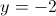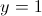# High School Math : Understanding Asymptotes

## Example Questions

2 Next →

### Example Question #1311 : High School Math

Find the vertical asymptote(s) of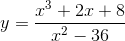.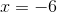and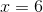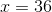There are no real vertical asymptotes.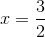andExplanation:

To find the vertical asymptotes, we set the denominator of the fraction equal to zero, as dividing anything by zero is "undefined." Since it's undefined, there's no way for us to graph that point!

Take our given equation,, and now set the denominator equal to zero: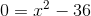.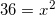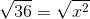Don't forget, the root of a positive number can be both positive or negative (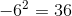as does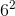), so our answer will be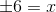.

Therefore the vertical asymptotes are atand.

### Example Question #21 : Exponents

Find the horizontal asymptote(s) of.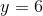and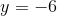There are no real horizontal asymptotes.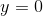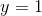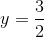There are no real horizontal asymptotes.

Explanation:

To find the horizontal asymptote of the function, look at the variable with the highest exponent. In the case of our equation,, the highest exponent is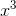in the numerator.

When the variable with the highest exponent is in the numberator, there are NO horizontal asymptotes. Horizontal asymptotes only appear when the greatest exponent is in the denominator OR when the exponents have same power in both the denominator and numerator.

### Example Question #1 : Quadratic Functions

What are the-intercepts of the equation?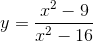There are no-intercepts.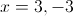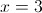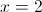Explanation:

To find the x-intercepts of the equation, we set the numerator equal to zero.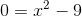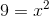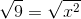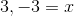### Example Question #1 : Solving And Graphing Exponential Equations

Find the vertical asymptote of the equation.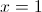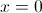There are no vertical asymptotes.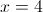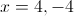Explanation:

To find the vertical asymptotes, we set the denominator of the function equal to zero and solve.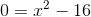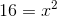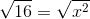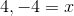### Example Question #14 : Solving And Graphing Exponential Equations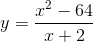What is the horizontal asymptote of this equation?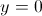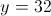There is no horizontal asymptote.